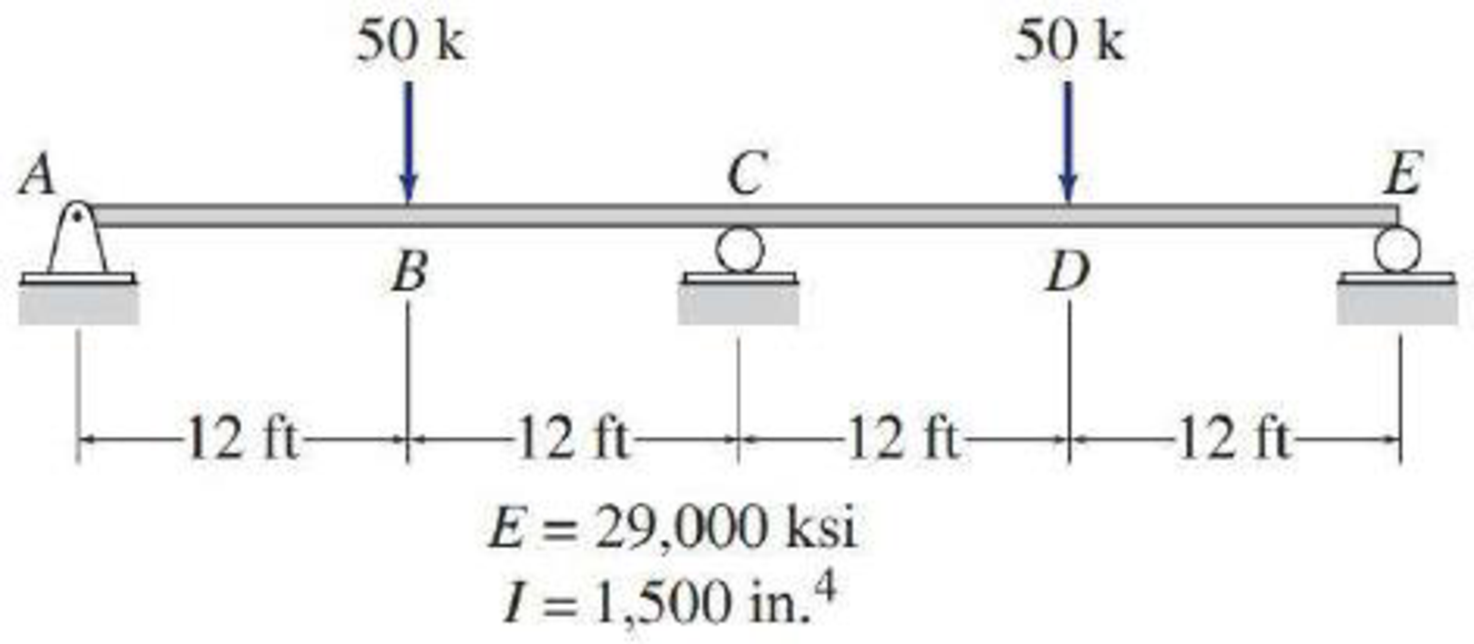# 13.9 through 13.12 Determine the reactions and draw the shear and bending moment diagrams for the beams shown in Figs. P13.9–P13.12 using the method of consistent deformations. Select the reaction at the interior support to be the redundant. FIG. P13.9, P13.30, P13.50

#### Solutions

Chapter
Section
Chapter 13, Problem 9P
Textbook Problem
171 views

## 13.9 through 13.12 Determine the reactions and draw the shear and bending moment diagrams for the beams shown in Figs. P13.9–P13.12 using the method of consistent deformations. Select the reaction at the interior support to be the redundant.FIG. P13.9, P13.30, P13.50To determine

Find the reactions and sketch the shear and bending moment diagrams for the given beam using method of consistent deformation.

### Explanation of Solution

Given information:

Apply the sign conventions for calculating reactions, forces and moments using the three equations of equilibrium as shown below.

• For summation of forces along x-direction is equal to zero (Fx=0), consider the forces acting towards right side as positive (+) and the forces acting towards left side as negative ().
• For summation of forces along y-direction is equal to zero (Fy=0), consider the upward force as positive (+) and the downward force as negative ().
• For summation of moment about a point is equal to zero (Matapoint=0), consider the clockwise moment as negative and the counter clockwise moment as positive.

Calculation:

Show the beam as shown in the Figure 1.

Refer Figure 1.

Consider the horizontal and vertical reaction at A are denoted by Ax and Ay.

Consider the moment at C is denoted by MC.

Consider the vertical reaction at C and E is denoted by Cy and Ey.

Find the degree of indeterminacy (DI).

The reactions (R) acting in the beam is 4.

The number of Equilibrium equation (E) is 3.

DI=RE=43=1

Take the vertical reaction at C as the redundant.

Modify the Figure 1 as shown in Figure 2.

Refer Figure 2.

The deflection at C due to the external force is denoted by ΔCO.

The deflection coefficient representing the deflection at C due to unit value of redundant Cy is fCC.

The deflection at C due to unknown redundant Cy is fCCCy.

Show the deflection at the mid of the beam due to the action of two point loads P, each acting at a distance of a from the supports as follows:

Δ=Pab3lEI(l2b2a2)        (1)

Here, l is the length of the beam, E is modulus of Elasticity of the beam, b is the distance between the loads, and I is the moment of inertia.

Find the deflection at the free end of the beam due to the load of 50 k as follows:

Substitute 12ft for a, 24ft for b, 48ft for l, and 50k for P in Equation (1).

ΔCO=50×12×243×48×EI(482242122)=158400kft3EI

Calculate the value of fCC as follows:

Show the deflection at the mid of the beam due to the action of central point load P as follows:

Δ2=Pl348EI        (2)

Substitute 1k for P and 48ft for l in Equation (2).

fCC=48348EI=2304kft3/kEI        (3)

Show the compatibility Equation as follows:

ΔOC+fCC×Cy=0

Modify the above Equation using Equation (1) and (3).

ΔCO+fCC×Cy=0158400kft3EI+2304kft3/kEI×Cy=0Cy=(EI2304kft3/k×158400kft3EI)Cy=68.75k()

Refer Figure 1.

Take the sum of the moment at A as zero.

MA=0(50×12)+Cy×24+Ey×48(50×36)=02400+Cy×24+Ey×48=0

Substitute 68

### Still sussing out bartleby?

Check out a sample textbook solution.

See a sample solution

#### The Solution to Your Study Problems

Bartleby provides explanations to thousands of textbook problems written by our experts, many with advanced degrees!

Get Started

Find more solutions based on key concepts
Select a rectangular (not square) HSS of A590 Grade C steel. a. Use LRFD. b. Use ASD.

Steel Design (Activate Learning with these NEW titles from Engineering!)

Present Example 11.5 in Chapter 11 using the format discussed in Section 4.2. Divide the example problem into G...

Engineering Fundamentals: An Introduction to Engineering (MindTap Course List)

Which paper is the foundation of all subsequent studies of computer security?

Principles of Information Security (MindTap Course List)

Derive the expression for the largest angle ? for which the homogeneous block stays in equilibrium.

International Edition---engineering Mechanics: Statics, 4th Edition

What is manufacturing?

Precision Machining Technology (MindTap Course List)

Internet Access Unavailable You are using a cloud storage provider to save files you want to use both at work a...

Enhanced Discovering Computers 2017 (Shelly Cashman Series) (MindTap Course List)

Explain the difference between a LAN and a WAN.

Systems Analysis and Design (Shelly Cashman Series) (MindTap Course List)

If your motherboard supports ECC DDR3 memory, can you substitute non-ECC DDR3 memory?

A+ Guide to Hardware (Standalone Book) (MindTap Course List)

What practical knowledge subject areas are covered in Module 1 of the SENSE program?

Welding: Principles and Applications (MindTap Course List)